•5星
389KB qq_44625365 2021-08-23 17:20:14
• 借助Excel TREND 函数来解决线性插值计算时间:2016-05-18作者:snow来源:互联网看题目感觉好高深，其实在工作中经常遇到，很实用的。但由于使用的函数比较冷门，会计算插值的同学很少。例：如下图所示，A列是数量，...

借助Excel TREND 函数来解决线性插值的计算

时间:2016-05-18   作者:snow   来源:互联网

看题目感觉好高深，其实在工作中经常遇到，很实用的。但由于使用的函数比较冷门，会计算插值的同学很少。

例：如下图所示，A列是数量，B列是数量对应的系数值。现要求出数字8所对应的系数值。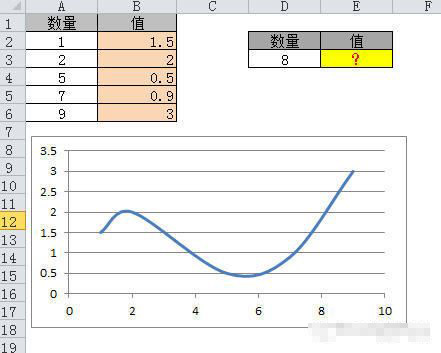分析：由于A列缺少值8，所以无法直接用excel函数计算出对应的值。今天我们要借助TREND函数来解决插值的计算。

TRAND函数，帮助里说它可以返回线性拟合线的一组纵坐标值，同学们只需要记得插值用TRAND函数就可以了。

TRAND(Y值数组,X值数组,X值)

注：X值数组要升序排列

本例中，D3单元格中的X值是8，因为它是在表中位于7~9之间，所以Y值数组应该是B5:B6，X值数组是A5:A6。

计算插值的公式为：

=TREND(B5:B6,A5:A6,D3) 结果为 1.95

如果D3值是4, Y值数组为：B3:B4，X值数组是A3:A4，计算插入的公式应修改为：

=TREND(B3:B4,A3:A4,D3) 计算结果为1

要是每算一个数，公式就要改一次，实在是太麻烦了。所以我们可以用match函数查找指定的X值在A列所对应的行数，然后用offset函数生成X和Y值的数组区域。即：

Y值区域

=OFFSET(B1,MATCH(D3,A2:A6,1),,2,1)

X值区域

=OFFSET(A1,MATCH(D3,A2:A6,1),,2,1)

把Y值区域和X值区域套进公式，即生成万能的插值公式：

=TREND(OFFSET(B1,MATCH(D3,A2:A6,1),,2,1),OFFSET(A1,MATCH(D3,A2:A6,1),,2,1),D3)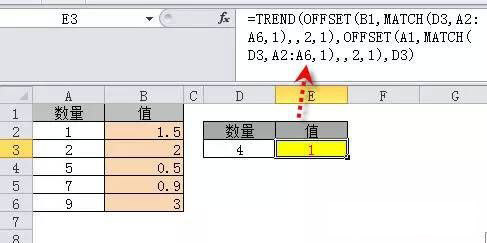本例的公式新手看起来可能有些吃力，同学们可以收藏一下本篇文章，以便将来套用和学习

展开全文weixin_33272515 2021-05-08 20:10:55
• python使用双线性插值计算网格内数据 python 双线性插值

最近在处理再分析资料网格数据时，需要用到插值方法，双线性插值速度比较快，所以考虑双线性插值方法。 双线性插值，故名思义就是在x和y方向分别进行线性插值。双线性插值一般用于图像处理，对一个个像素块进行线性...

最近在处理再分析资料网格数据时，需要用到插值方法，双线性插值速度比较快，所以考虑双线性插值方法。

双线性插值，故名思义就是在x和y方向分别进行线性插值。双线性插值一般用于图像处理，对一个个像素块进行线性插值。

1.双线性插值

已知网格点Q12，Q22，Q11，Q21，但是要插值的点为P点，这就要用双线性插值了，首先在x轴方向上，对R1和R2两个点进行插值，这个很简单，然后根据R1和R2对P点进行插值，这就是所谓的双线性插值。在数学上，双线性插值是有两个变量的插值函数的线性插值扩展，其核心思想是在两个方向分别进行一次线性插值。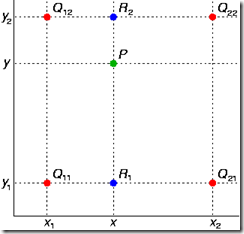假如我们想得到未知函数 f 在点 P = (x, y) 的值，假设我们已知函数 f 在 Q11 = (x1, y1)、Q12 = (x1, y2), Q21 = (x2, y1) 以及 Q22 = (x2, y2) 四个点的值。

1） 在x方向上进行线性插值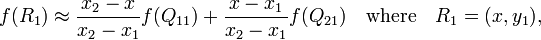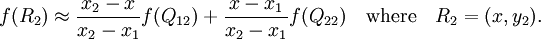2） 在y方向上进行线性插值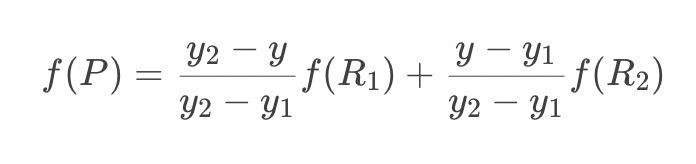3） 综合起来，就是双线性插值的结果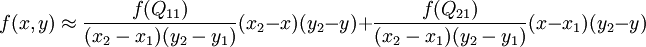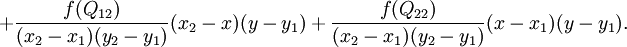其中(x2 - x1)， (y2 - y1) 为网格间距。这样只需要根据网格点上的值，网格间距，以及网格内需要插值的点的位置就可以计算插值结果。

2.python实现双线性插值

一般使用的再分析资料如NCEP，分辨率为1° x 1°的，网格间距即为1，则(x2 - x1)， (y2 - y1)都为1，

则上面的公式分母为1，结果为：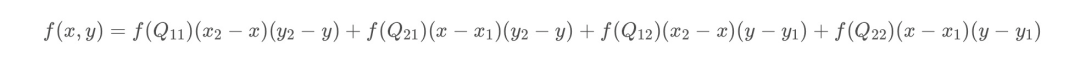首先要根据经纬度计算网格点上的值，这里为计算网格点上的风速：

# time为时间间隔，lat,lon为网格点的经纬度，转化为索引，提取u10和v10的数据，计算风速
def Cal_wind(time, lat, lon):
u_wind = nc_obj.variables['u10'][time][lat_index(lat)][lon_index(lon)]
v_wind = nc_obj.variables['v10'][time][lat_index(lat)][lon_index(lon)]
wind = math.sqrt(math.pow(u_wind, 2) + math.pow(v_wind, 2))
return wind

然后根据插值的经纬度，以及它所在网格的四个经纬度，实现双线性插值：

# x,y为插值点的经纬度，x1为网格左经度，x2为网格右经度，y1为网格下纬度，y2为网格上纬度,输出双线性插值的结果
def bi_linear(x, y, x1, y1, x2, y2, time):

wind11 = Cal_wind(time, y1, x1)  # 网格左下角的风速
wind12 = Cal_wind(time, y2, x1)  # 网格左上角的风速
wind21 = Cal_wind(time, y1, x2)  # 网格右下角的风速
wind22 = Cal_wind(time, y2, x2)  # 网格右上角的风速
print(wind11, wind12, wind21, wind22)

wind_bi_linear_interp = (1 / ((x2 - x1) * (y2 - y1))) * (wind11 * (x2 - x) * (y2 - y) + wind21 * (x - x1) * (y2 - y) + wind12 * (x2 - x) * (y - y1) + wind22 * (x - x1) * (y - y1))
return wind_bi_linear_interp

展开全文weixin_44052055 2021-08-23 09:42:34
• python插值计算 python

一维插值 常规用法 【参考文章】 python实现各种插值法(数值分析) python scipy样条插值函数大全（interpolate里interpld函数） Python：插值interpolate模块 示例代码 # -*-coding:utf-8 -*- import numpy as np ...

一维插值

常规用法

# -*-coding:utf-8 -*-
import numpy as np
from scipy import interpolate
import pylab as pl

x=np.linspace(0,10,11)
#x=[ 0. 1. 2. 3. 4. 5. 6. 7. 8. 9. 10.]
y=np.sin(x)
xnew=np.linspace(0,10,101)
pl.plot(x,y,"ro")

#"nearest","zero"为阶梯插值
#slinear 线性插值
f=interpolate.interp1d(x,y,kind=kind)
# ‘slinear', ‘quadratic' and ‘cubic' refer to a spline interpolation of first, second or third order)
ynew=f(xnew)
pl.plot(xnew,ynew,label=str(kind))
pl.legend(loc="lower right")
pl.show()

处理外推

当插值超出范围的时候如果不加处理会导致报错，这时有如下几个方案。
【参考文章】
插值超出Python范围
如何使scipy.interpolate给出超出输入范围的外推结果？

在interpolate下设置bounds_error和fill_value

bounds_error：布尔值，可选
如果为True，则任何时候尝试对x范围之外的值进行插值都会引发ValueError（需要进行插值）。如果为False，则为边界值分配fill_value。fill_value默认情况下返回Nan，如果采用外推则设置fill_value=“extrapolate”。

import numpy as np
from scipy import interpolate

x = np.arange(0,10)
y = np.exp(-x/3.0)
f = interpolate.interp1d(x, y, bounds_error=False,fill_value="extrapolate")

print(f(9),np.exp(-9/3),f(9)-np.exp(-9/3))
#0.049787068367863944 0.049787068367863944 0.0
print(f(11),np.exp(-11/3),f(11)-np.exp(-11/3))
#0.049787068367863944 0.025561533206507402 0.024225535161356542

由于外推部分是线性外推，所以对于非线性函数会有些许误差。
当然fill_value也可以是具体的值，比如0，或者边界值y[-1]（但要注意设置边界值的话可能出现左侧溢出却返回右侧边界值的情况，此时应做相应处理，或者采用下面的interp函数。

采用interp

interp是将左侧和右侧的边界值直接作为超出范围的部分，而且使用格式与interpolate有些不同

import numpy as np
from scipy import interp

x = np.arange(0,10)
y = np.exp(-x/3.0)
interp([9,10],x, y)  #两个插值返回结果相同

单变量插值UnivariateSpline

参考文章 Python：插值interpolate模块
UnivariateSpline可以外插值
调用方式如下：

UnivariateSpline(x,y,w=None,bbox=[None,None],k=3,s=None)

• x,y是X-Y坐标数组
• w是每个数据点的权重值
• k为样条曲线的阶数
• s为平滑参数。
• s=0，样条曲线强制通过所有数据点
• s>0,满足 ∑ ( w ( y − spline ⁡ ( x ) ) ) 2 ≤ s \sum(w(y-\operatorname{spline}(x)))^{2} \leq s

s=0强制通过所有数据点的外插值

from scipy import interpolate
import numpy as np
x1=np.linspace(0,10,20)
y1=np.sin(x1)

sx1=np.linspace(0,12,100)
func1=interpolate.UnivariateSpline(x1,y1,s=0)#强制通过所有点
sy1=func1(sx1)

import matplotlib.pyplot as plt
plt.plot(x1,y1,'o')
plt.plot(sx1,sy1)
plt.show()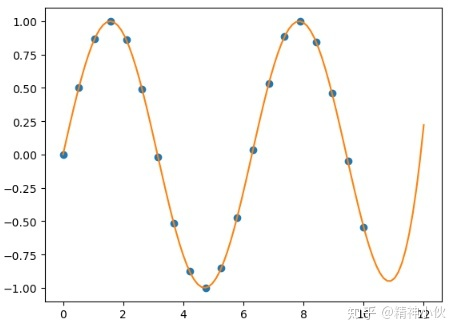也就插值到(0,12)，范围再大就不行了，毕竟插值的专长不在于预测

s>0：不强制通过所有点

import numpy as np
from scipy import interpolate
x2=np.linspace(0,20,200)
y2=np.sin(x2)+np.random.normal(loc=0,scale=1,size=len(x2))*0.2

sx2=np.linspace(0,22,2000)
func2=interpolate.UnivariateSpline(x2,y2,s=8)
sy2=func2(sx2)

import matplotlib.pyplot as plt
plt.plot(x2,y2,'.')
plt.plot(sx2,sy2)
plt.show()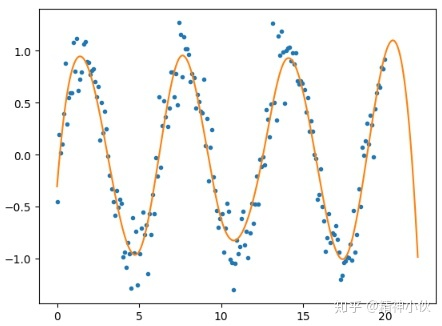多维插值

二维插值仍然参考文章 如何使scipy.interpolate给出超出输入范围的外推结果？
使用的函数为interp2d()

interp2d(x,y,z,kind='linear')

这里有几个注意事项：

• interp2d()中，输入的x,y,z先用ravel()被转成了一维数组
• func()的输入必须是一维的，输出是二维的（有点奇怪，感觉完成度不高）。但如果是点插值，而不是直接一个网格插值，那这一点没什么影响。
• 插值的源数据必须是等距网格。不然的haul，运行不保存但结果不对。

step1:生成数据

import numpy as np
def func(x,y):
return (x+y)*np.exp(-5*(x**2+y**2))
x,y=np.mgrid[-1:1:8j,-1:1:8j]
z=func(x,y)

step2:插值

from scipy import interpolate
func=interpolate.interp2d(x,y,z,kind='cubic')

xnew=np.linspace(-1,1,100)
ynew=np.linspace(-1,1,100)
znew=func(xnew,ynew)#xnew, ynew是一维的，输出znew是二维的
xnew,ynew=np.mgrid[-1:1:100j,-1:1:100j]#统一变成二维，便于下一步画图

step3:画图

import mpl_toolkits.mplot3d
import matplotlib.pyplot as plt
ax=plt.subplot(111,projection='3d')
ax.plot_surface(xnew,ynew,znew)
ax.scatter(x,y,z,c='r',marker='^')
plt.show()

4.二维插值Rbf()
Rbf的优点是，排列可以无序，可以不是等距的网格

step1:随机生成点，并计算函数值

import numpy as np
def func(x,y):
return (x+y)*np.exp(-5*(x**2+y**2))

x=np.random.uniform(low=-1,high=1,size=100)
y=np.random.uniform(low=-1,high=1,size=100)
z=func(x,y)

step2：插值

from scipy import  interpolate
xnew,ynew=np.mgrid[-1:1:100j,-1:1:100j]
znew=func(xnew,ynew)#输入输出都是二维

step3：画图

import mpl_toolkits.mplot3d
import matplotlib.pyplot as plt
ax=plt.subplot(111,projection='3d')
ax.plot_surface(xnew,ynew,znew)
ax.scatter(x,y,z,c='r',marker='^')
plt.show()

展开全文gsgbgxp 2021-04-26 10:43:01
•   在图像的仿射变换中，很多地方需要用到插值运算，常见的插值运算包括最邻近插值，双线性插值，双三次插值，兰索思插值等方法，OpenCV提供了很多方法，其中，双线性插值由于折中的插值效果和运算速度，运用比较...

引出

在图像的仿射变换中，很多地方需要用到插值运算，常见的插值运算包括最邻近插值，双线性插值，双三次插值，兰索思插值等方法，OpenCV提供了很多方法，其中，双线性插值由于折中的插值效果和运算速度，运用比较广泛。
越是简单的模型越适合用来举例子，我们就举个简单的图像：3*3 的256级灰度图。假如图像的象素矩阵如下图所示（这个原始图把它叫做源图，Source）：

234 38  22
67  44  12
89  65  63

这个矩阵中，元素坐标(x,y)是这样确定的，x从左到右，从0开始，y从上到下，也是从零开始，这是图象处理中最常用的坐标系。
如果想把这副图放大为 4*4大小的图像，那么该怎么做呢？那么第一步肯定想到的是先把4*4的矩阵先画出来再说，好了矩阵画出来了，如下所示，当然，矩阵的每个像素都是未知数，等待着我们去填充（这个将要被填充的图的叫做目标图,Destination）：

? ? ? ?
? ? ? ?
? ? ? ?
? ? ? ?

然后要往这个空的矩阵里面填值了，要填的值从哪里来来呢？是从源图中来，好，先填写目标图最左上角的象素，坐标为（0，0），那么该坐标对应源图中的坐标可以由如下公式得出srcX=dstX* (srcWidth/dstWidth) , srcY = dstY * (srcHeight/dstHeight)
好了，套用公式，就可以找到对应的原图的坐标了(0*(3/4),0*(3/4))=>(0*0.75,0*0.75)=>(0,0)，找到了源图的对应坐标,就可以把源图中坐标为(0,0)处的234象素值填进去目标图的(0,0)这个位置了。
接下来,如法炮制,寻找目标图中坐标为(1,0)的象素对应源图中的坐标,套用公式:
(1*0.75,0*0.75)=>(0.75,0) 结果发现,得到的坐标里面竟然有小数,这可怎么办?计算机里的图像可是数字图像,象素就是最小单位了,象素的坐标都是整数,从来没有小数坐标。这时候采用的一种策略就是采用四舍五入的方法（也可以采用直接舍掉小数位的方法），把非整数坐标转换成整数，好，那么按照四舍五入的方法就得到坐标（1，0），完整的运算过程就是这样的：(1*0.75,0*0.75)=>(0.75,0)=>(1,0) 那么就可以再填一个象素到目标矩阵中了，同样是把源图中坐标为(1,0)处的像素值38填入目标图中的坐标。
依次填完每个象素，一幅放大后的图像就诞生了，像素矩阵如下所示：

234 38  22  22
67  44  12  12
89  65  63  63
89  65  63  63

这种放大图像的方法叫做最临近插值算法，这是一种最基本、最简单的图像缩放算法，效果也是最不好的，放大后的图像有很严重的马赛克，缩小后的图像有很严重的失真；效果不好的根源就是其简单的最临近插值方法引入了严重的图像失真，比如，当由目标图的坐标反推得到的源图的的坐标是一个浮点数的时候，采用了四舍五入的方法，直接采用了和这个浮点数最接近的象素的值，这种方法是很不科学的，当推得坐标值为 0.75的时候，不应该就简单的取为1，既然是0.75，比1要小0.25 ，比0要大0.75 ,那么目标象素值其实应该根据这个源图中虚拟的点四周的四个真实的点来按照一定的规律计算出来的，这样才能达到更好的缩放效果。
双线型内插值算法就是一种比较好的图像缩放算法，它充分的利用了源图中虚拟点四周的四个真实存在的像素值来共同决定目标图中的一个像素值，因此缩放效果比简单的最邻近插值要好很多。

双线性内插值算法描述

对于一个目的像素，设置坐标通过反向变换得到的浮点坐标为(i+u,j+v) (其中i、j均为浮点坐标的整数部分，u、v为浮点坐标的小数部分，是取值[0,1)区间的浮点数)，则这个像素得值 f(i+u,j+v) 可由原图像中坐标为 (i,j)、(i+1,j)、(i,j+1)、(i+1,j+1)所对应的周围四个像素的值决定，即：
f(i+u,j+v) = (1-u)(1-v)f(i,j) + (1-u)vf(i,j+1) + u(1-v)f(i+1,j) + uvf(i+1,j+1)
其中f(i,j)表示源图像(i,j)处的的像素值，以此类推。
比如，像刚才的例子，现在假如目标图的象素坐标为（1，1），那么反推得到的对应于源图的坐标是（0.75 , 0.75）, 这其实只是一个概念上的虚拟象素,实际在源图中并不存在这样一个象素,那么目标图的象素（1，1）的取值不能够由这个虚拟象素来决定，而只能由源图的这四个象素共同决定：（0，0）（0，1）（1，0）（1，1），而由于（0.75,0.75）离（1，1）要更近一些，那么（1,1）所起的决定作用更大一些，这从公式1中的系数uv=0.75×0.75就可以体现出来，而（0.75,0.75）离（0，0）最远，所以（0，0）所起的决定作用就要小一些，公式中系数为(1-u)(1-v)=0.25×0.25也体现出了这一特点。

计算方法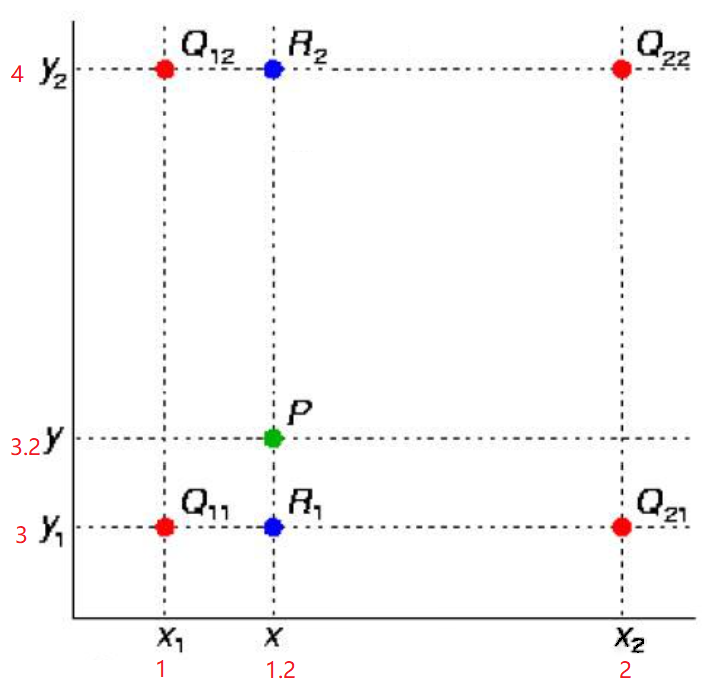首先，在X方向上进行两次线性插值计算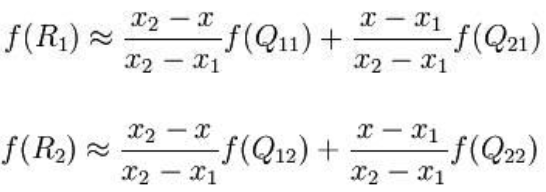然后在Y方向上进行一次插值计算：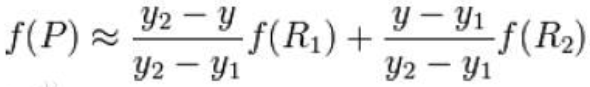在图像处理的时候，我们先根据

srcX = dstX * (srcWidth/dstWidth),
srcY = dstY * (srcHeight/dstHeight)

来计算目标像素在源图像中的位置，这里计算的srcX和srcY一般都是浮点数，比如f（1.2, 3.2）这个像素点是虚拟存在的，先找到与它临近的四个实际存在的像素点：

（1，4） （2，4）
（1，3） （2，3）

写成f(i+u,j+v)的形式，则u=0.2,v=0.2, i=1, j=3
假设他们的值为：

100 200
300 400

根据上面公式：

x1=1  x=1.2  x2=2
y1=3  y=3.2  y2=4
f(Q12)=100  f(Q22)=200
f(Q11)=300  f(Q21)=400

f(R1)=( (2-1.2)/(2-1) )*300 + ( (1.2-1)/(2-1) )*400 = 0.8*300 + 0.2*400 = 320
f(R2)=( (2-1.2)/(2-1) )*100 + ( (1.2-1)/(2-1) )*200 = 0.8*100 + 0.2*200 = 120

f(P)=( (4-3.2)/(4-3) )*f(R1) + ( (3.2-3)/(4-3) )*f(R2) = 0.8*320 + 0.2*120 = 280

也可以一步直接计算：
f(i+u,j+v) = (1-u)(1-v)f(i,j) + (1-u)vf(i,j+1) + u(1-v)f(i+1,j) + uvf(i+1,j+1)

加速以及优化策略

单纯按照上文实现的插值算法只能勉强完成插值的功能，速度和效果都不会理想，在具体代码实现的时候有些小技巧。参考OpenCV源码以及网上博客整理如下两点：

• 源图像和目标图像几何中心的对齐。
• 将浮点运算转换成整数运算

源图像和目标图像几何中心的对齐

在计算源图像的虚拟浮点坐标的时候，一般情况：

srcX = dstX * (srcWidth/dstWidth) ,
srcY = dstY * (srcHeight/dstHeight)

中心对齐(OpenCV也是如此)：

SrcX = (dstX+0.5) * (srcWidth/dstWidth) - 0.5
SrcY = (dstY+0.5) * (srcHeight/dstHeight) - 0.5

原理：
将公式变形：

srcX=dstX* (srcWidth/dstWidth)+0.5*(srcWidth/dstWidth-1)

相当于我们在原始的浮点坐标上加上了0.5*(srcWidth/dstWidth-1)这样一个控制因子，这项的符号可正可负，与srcWidth/dstWidth的比值也就是当前插值是扩大还是缩小图像有关，有什么作用呢？看一个例子：
假设源图像是3*3，中心点坐标（1，1），目标图像是9*9，中心点坐标（4，4），我们在进行插值映射的时候，尽可能希望均匀的用到源图像的像素信息，最直观的就是（4,4）映射到（1,1），现在直接计算srcX=4*(3/9)=1.3333 != 1，也就是我们在插值的时候所利用的像素集中在图像的右下方，而不是均匀分布整个图像。现在考虑中心点对齐，srcX=(4+0.5)*3/9-0.5=1，刚好满足我们的要求。

将浮点运算转换成整数运算

直接进行计算的话，由于计算的srcX和srcY 都是浮点数，后续会进行大量的乘法，而图像数据量又大，速度不会理想，解决思路是：
浮点运算→→整数运算→→”<<左右移按位运算”。

放大的主要对象是u，v这些浮点数，OpenCV选择的放大倍数是2048“如何取这个合适的放大倍数呢，要从三个方面考虑：

• 第一：精度问题，如果这个数取得过小，那么经过计算后可能会导致结果出现较大的误差。
• 第二，这个数不能太大，太大会导致计算过程超过长整形所能表达的范围。
• 第三：速度考虑。假如放大倍数取为12，那么算式在最后的结果中应该需要除以12*12=144，但是如果取为16，则最后的除数为16*16=256，这个数字好，我们可以用右移来实现，而右移要比普通的整除快多了。”我们利用左移11位操作就可以达到放大目的。

opencv代码

cv::Mat matSrc, matDst1, matDst2;

matSrc = cv::imread("lena.jpg", 2 | 4);
matDst1 = cv::Mat(cv::Size(800, 1000), matSrc.type(), cv::Scalar::all(0));
matDst2 = cv::Mat(matDst1.size(), matSrc.type(), cv::Scalar::all(0));

double scale_x = (double)matSrc.cols / matDst1.cols;
double scale_y = (double)matSrc.rows / matDst1.rows;

int stepDst = matDst1.step;
uchar* dataSrc = matSrc.data;
int stepSrc = matSrc.step;
int iWidthSrc = matSrc.cols;
int iHiehgtSrc = matSrc.rows;

for (int j = 0; j < matDst1.rows; ++j)
{
float fy = (float)((j + 0.5) * scale_y - 0.5);
int sy = cvFloor(fy);
fy -= sy;
sy = std::min(sy, iHiehgtSrc - 2);
sy = std::max(0, sy);

short cbufy;
cbufy = cv::saturate_cast<short>((1.f - fy) * 2048);
cbufy = 2048 - cbufy;

for (int i = 0; i < matDst1.cols; ++i)
{
float fx = (float)((i + 0.5) * scale_x - 0.5);
int sx = cvFloor(fx);
fx -= sx;

if (sx < 0) {
fx = 0, sx = 0;
}
if (sx >= iWidthSrc - 1) {
fx = 0, sx = iWidthSrc - 2;
}

short cbufx;
cbufx = cv::saturate_cast<short>((1.f - fx) * 2048);
cbufx = 2048 - cbufx;

for (int k = 0; k < matSrc.channels(); ++k)
{
*(dataDst+ j*stepDst + 3*i + k) = (*(dataSrc + sy*stepSrc + 3*sx + k) * cbufx * cbufy +
*(dataSrc + (sy+1)*stepSrc + 3*sx + k) * cbufx * cbufy +
*(dataSrc + sy*stepSrc + 3*(sx+1) + k) * cbufx * cbufy +
*(dataSrc + (sy+1)*stepSrc + 3*(sx+1) + k) * cbufx * cbufy) >> 22;
}
}
}
cv::imwrite("linear_1.jpg", matDst1);

cv::resize(matSrc, matDst2, matDst1.size(), 0, 0, 1);
cv::imwrite("linear_2.jpg", matDst2);

展开全文qlexcel 2021-08-01 15:20:04
• weixin_31577315 2021-01-17 13:07:40
• 利用MatLab对数据进行插值计算（分段插值和三次样条插值） matlab

APANGG123 2021-07-04 20:32:40
• 插值法怎么计算，完全看不懂 js插值计算

weixin_34929072 2020-12-29 06:41:51
• weixin_28829903 2021-04-23 09:52:23
• weixin_30003217 2021-03-15 01:07:06
• 信号的插值计算 算法

STR618 2021-10-25 21:49:48
• xingzhan2142 2021-06-26 12:29:30
• weixin_32017023 2021-01-17 10:24:12
• weixin_39888807 2020-12-21 17:11:17
• [Matlab科学计算] 使用Kriging工具箱进行简单的插值计算 Kriging插值 matlab

zhwzhaowei 2021-03-03 11:15:58
• XY坐标两点插值（加密）计算（Java） java 算法 插值算法

LXDOS 2021-10-19 10:06:49
• 计算方法（五）函数插值 数学

m0_47704575 2021-05-23 14:56:50
• 数值计算之 插值法（6）样条插值 线性代数 矩阵 机器学习

qq_41035283 2021-11-21 20:05:12
• weixin_39788703 2021-06-22 23:19:51
• 数值计算之 插值法（2）多项式插值——牛顿插值法 线性代数 矩阵

qq_41035283 2021-11-17 15:30:42
• 数值计算之 插值法（1）多项式插值——拉格朗日插值法 线性代数 矩阵

qq_41035283 2021-11-17 10:39:38
• 数值计算(二)——插值计算(1)拉格朗日插值法 拉格朗日插值 插值法 数值计算 数值分析

qq_41606378 2021-12-17 17:14:46
• weixin_39900468 2021-01-14 00:13:04
• weixin_36356002 2021-05-20 06:54:48
• 数值计算之 插值法（5）分段插值，埃尔米特插值 线性代数 矩阵 算法

qq_41035283 2021-11-20 16:51:35
• weixin_42405852 2021-04-21 06:33:39
• weixin_32957761 2021-03-13 09:00:21
• [计算机数值分析]拉格朗日插值公式 c++ 数值分析 拉格朗日插值公式

weixin_51008866 2021-04-20 21:10:50
• Vue学习笔记（一）——插值操作与计算属性 vue vue.js

qq2297329956 2021-01-26 11:43:24
• js图像处理 双线性插值 双三次插值法 实现 js插值计算

weixin_39887386 2020-12-19 20:55:53
• weixin_45720246 2021-04-27 08:34:56...